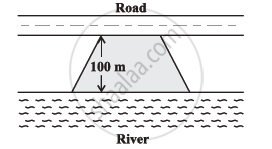Share

# Mohan Wants to Buy a Trapezium Shaped Field. Its Side Along the River is Parallel to and Twice the Side Along the Road. It the Area of this Field is 10500 M2 And the Perpendicular Distance Between the Two Parallel Sides is 100 M, Find the Length of the Side Along the River - CBSE Class 8 - Mathematics

ConceptArea of a Polygon

#### Question

Mohan wants to buy a trapezium shaped field. Its side along the river is parallel to and twice the side along the road. It the area of this field is 10500 m2 and the perpendicular distance between the two parallel sides is 100 m, find the length of the side along the river.#### Solution

Let the length of the field along the road be m. Hence, the length of the field along the river will be 2l m.

Area of trapezium = 1/2 (Sum of parallel sides) (Distance between the parallel sides)

=>10500 m^2 = 1/2 (l + 2l) xx (100 m)

3l = ((2 xx 10500)/100)m = 210 m

l = 70 m

Thus, length of the field along the river = (2 × 70) m = 140 m

Is there an error in this question or solution?

#### APPEARS IN

NCERT Solution for Mathematics Textbook for Class 8 (2018 to Current)
Chapter 11: Mensuration
Ex. 11.20 | Q: 8 | Page no. 178
Solution Mohan Wants to Buy a Trapezium Shaped Field. Its Side Along the River is Parallel to and Twice the Side Along the Road. It the Area of this Field is 10500 M2 And the Perpendicular Distance Between the Two Parallel Sides is 100 M, Find the Length of the Side Along the River Concept: Area of a Polygon.
S